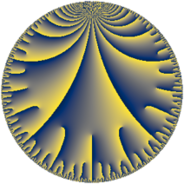# Properties

 Label 33.14.fLevel $33$ Weight $14$ Character orbit 33.f Rep. character $\chi_{33}(2,\cdot)$ Character field $\Q(\zeta_{10})$ Dimension $200$ Sturm bound $56$

# Related objects

## Defining parameters

 Level: $$N$$ $$=$$ $$33 = 3 \cdot 11$$ Weight: $$k$$ $$=$$ $$14$$ Character orbit: $$[\chi]$$ $$=$$ 33.f (of order $$10$$ and degree $$4$$) Character conductor: $$\operatorname{cond}(\chi)$$ $$=$$ $$33$$ Character field: $$\Q(\zeta_{10})$$ Sturm bound: $$56$$

## Dimensions

The following table gives the dimensions of various subspaces of $$M_{14}(33, [\chi])$$.

Total New Old
Modular forms 216 216 0
Cusp forms 200 200 0
Eisenstein series 16 16 0

## Trace form

 $$200 q + 858 q^{3} - 196614 q^{4} + 399675 q^{6} - 10 q^{7} - 1889918 q^{9} + O(q^{10})$$ $$200 q + 858 q^{3} - 196614 q^{4} + 399675 q^{6} - 10 q^{7} - 1889918 q^{9} - 32831586 q^{12} - 10 q^{13} - 140222862 q^{15} - 927970186 q^{16} - 488217285 q^{18} - 1437362980 q^{19} + 490978148 q^{22} - 4911226885 q^{24} + 11583962988 q^{25} - 2915991372 q^{27} - 30696089730 q^{28} + 16211506330 q^{30} - 7112670716 q^{31} - 12041033248 q^{33} + 48330088316 q^{34} + 23959456451 q^{36} - 40776243462 q^{37} - 75493012900 q^{39} + 138068623350 q^{40} + 12445598948 q^{42} - 3610101576 q^{45} - 79583947530 q^{46} + 311573919278 q^{48} + 324365200528 q^{49} - 126148257390 q^{51} + 218399483410 q^{52} + 1131283098400 q^{55} - 2226554718810 q^{57} - 3016799703178 q^{58} + 2183025503450 q^{60} + 2158021129430 q^{61} + 1807068500970 q^{63} - 3880841974954 q^{64} - 6732877864344 q^{66} - 787709909812 q^{67} + 3684632398376 q^{69} + 1927563776896 q^{70} - 16936910180840 q^{72} + 4469075247240 q^{73} + 4357015652442 q^{75} + 12455666975512 q^{78} + 15653822613410 q^{79} + 1045365851014 q^{81} - 1646931685984 q^{82} + 14486919340920 q^{84} + 19186751785210 q^{85} + 11538275958734 q^{88} - 17525050163960 q^{90} - 13637602974634 q^{91} + 21868314681346 q^{93} + 54963146902410 q^{94} - 85543078333310 q^{96} + 29368579014654 q^{97} + 47770951885072 q^{99} + O(q^{100})$$

## Decomposition of $$S_{14}^{\mathrm{new}}(33, [\chi])$$ into newform subspaces

The newforms in this space have not yet been added to the LMFDB.